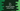# C++ program to find student grades using if-else### C++ program to find student grades using if-else:

In this post, we will learn how to find the grades of Students using if-else checks. It will take the marks for a user as input and using a if-else block, it will print the grade.

The program will take the marks for n number of subjects. Then it will find the average from these marks and print out the grade based on it.

Below is the algorithm that the program will use:

### Algorithm to find student grades:

• Take the total number of subjects from the user.
• Read marks for each of these subjects one by one.
• Find the average of all these marks.
• Using if-else, find the grade and print it out.

### C++ program:

Below is the complete C++ program:

``````#include <iostream>

using namespace std;

int main()
{

int totalSubjects;
int totalMarks = 0;
int avgMarks;
int currentMarks;

cout << "Enter total number of subjects :" << endl;
cin >> totalSubjects;

for (int i = 0; i < totalSubjects; i++)
{
cout << "Enter marks for subject " << i + 1 << " :" << endl;
cin >> currentMarks;
totalMarks += currentMarks;
}

avgMarks = totalMarks / totalSubjects;

{
cout << "Grade A" << endl;
}
{
cout << "Grade B" << endl;
}
{
cout << "Grade C" << endl;
}
else
{
cout << "Grade D" << endl;
}
}``````

### Explanation:

In this program :

2. We are reading the total subjects from the user and storing that value in totalSubjects.
3. Using a for loop, we are reading the marks of the user one by one. Each mark is stored in currentMarks and that marks is added to totalMarks.
4. The average marks, avgMarks is calculated by dividing the total marks by total subjects.
5. Finally, we are comparing this avgMarks with the grade constants and printing the grade for that marks.

### Sample Output:

``````Enter total number of subjects :
3
Enter marks for subject 1 :
40
Enter marks for subject 2 :
45
Enter marks for subject 3 :
56

Enter total number of subjects :
4
Enter marks for subject 1 :
89
Enter marks for subject 2 :
90
Enter marks for subject 3 :
93
Enter marks for subject 4 :
94